[Office] WPS Excel通过添加宏实现多张表格合并

一、合并当前文件夹下所有文件表格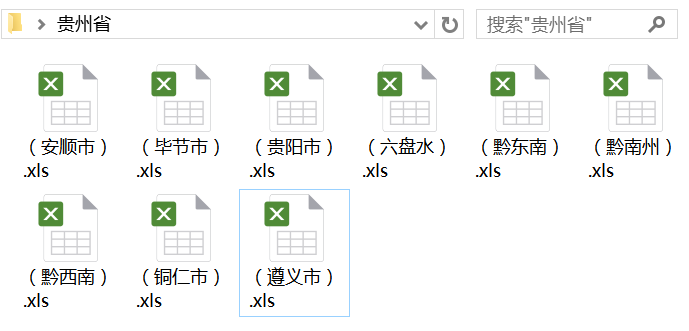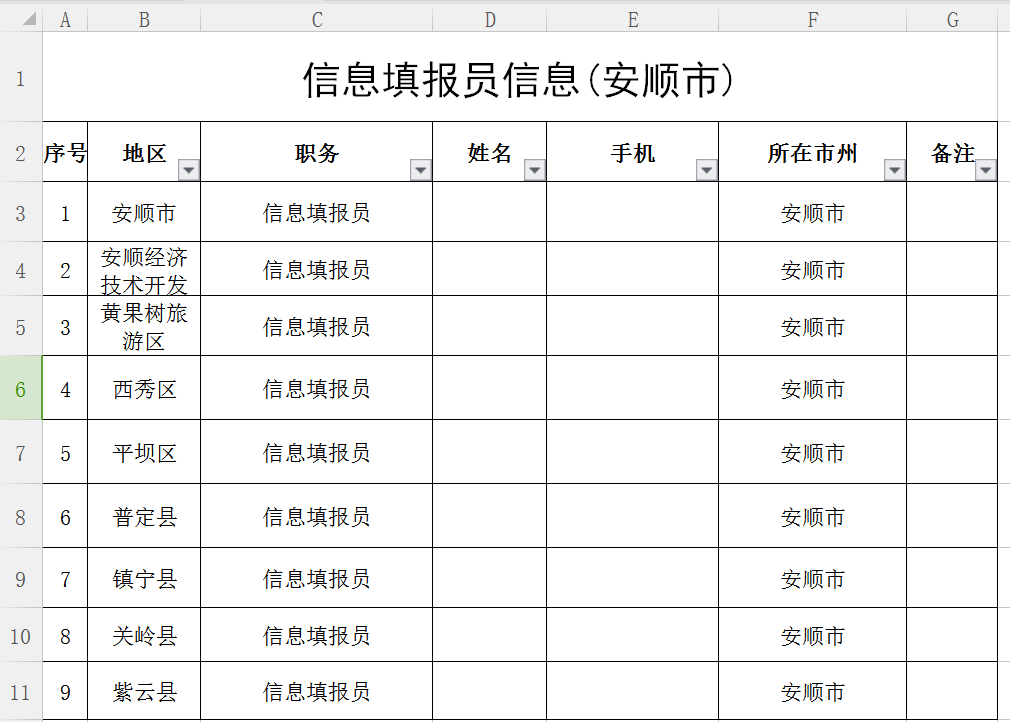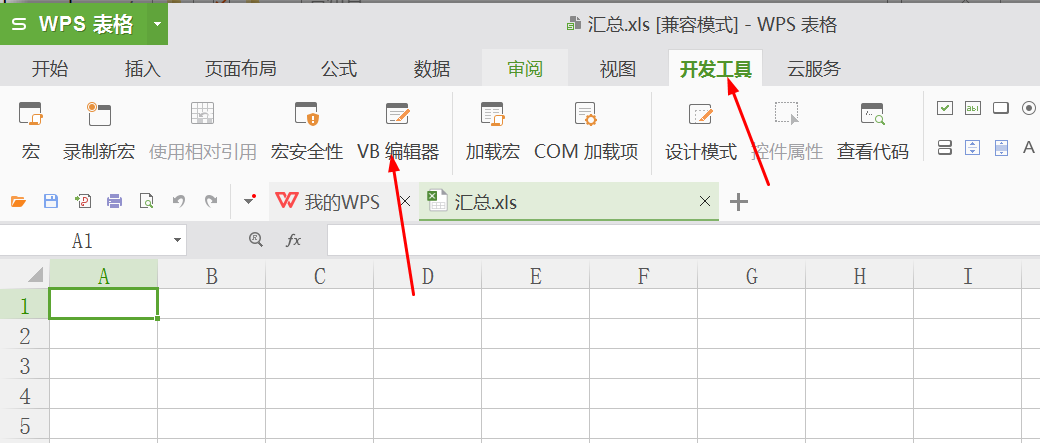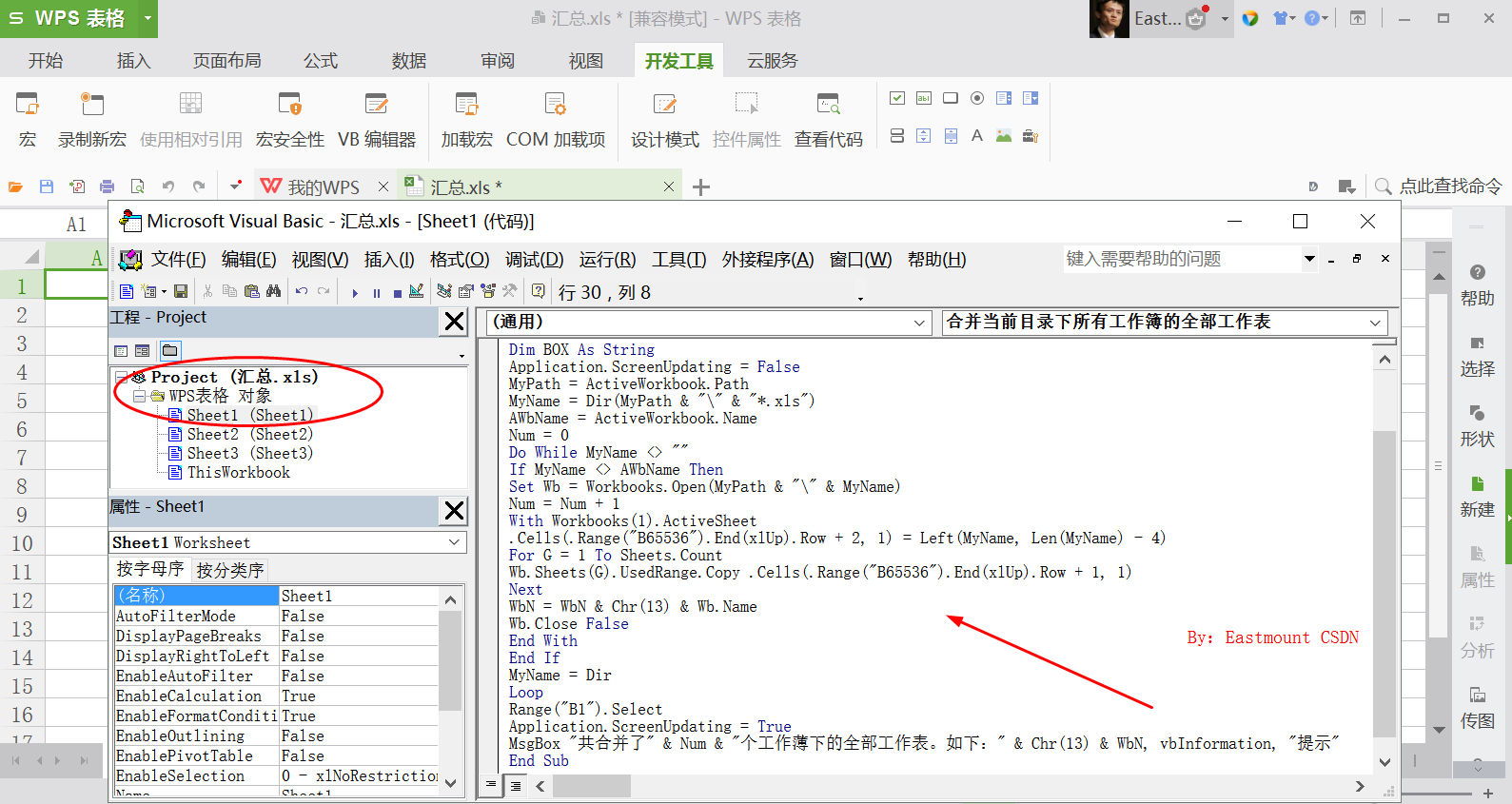Sub 合并当前目录下所有工作簿的全部工作表()
Dim MyPath, MyName, AWbName
Dim Wb As workbook, WbN As String
Dim G As Long
Dim Num As Long
Dim BOX As String
Application.ScreenUpdating = False
MyPath = ActiveWorkbook.Path
MyName = Dir(MyPath & "\" & "*.xls")
AWbName = ActiveWorkbook.Name
Num = 0
Do While MyName <> ""
If MyName <> AWbName Then
Set Wb = Workbooks.Open(MyPath & "\" & MyName)
Num = Num + 1
With Workbooks(1).ActiveSheet
.Cells(.Range("B65536").End(xlUp).Row + 2, 1) = Left(MyName, Len(MyName) - 4)
For G = 1 To Sheets.Count
Wb.Sheets(G).UsedRange.Copy .Cells(.Range("B65536").End(xlUp).Row + 1, 1)
Next
WbN = WbN & Chr(13) & Wb.Name
Wb.Close False
End With
End If
MyName = Dir
Loop
Range("B1").Select
Application.ScreenUpdating = True
MsgBox "共合并了" & Num & "个工作薄下的全部工作表。如下：" & Chr(13) & WbN, vbInformation, "提示"
End Sub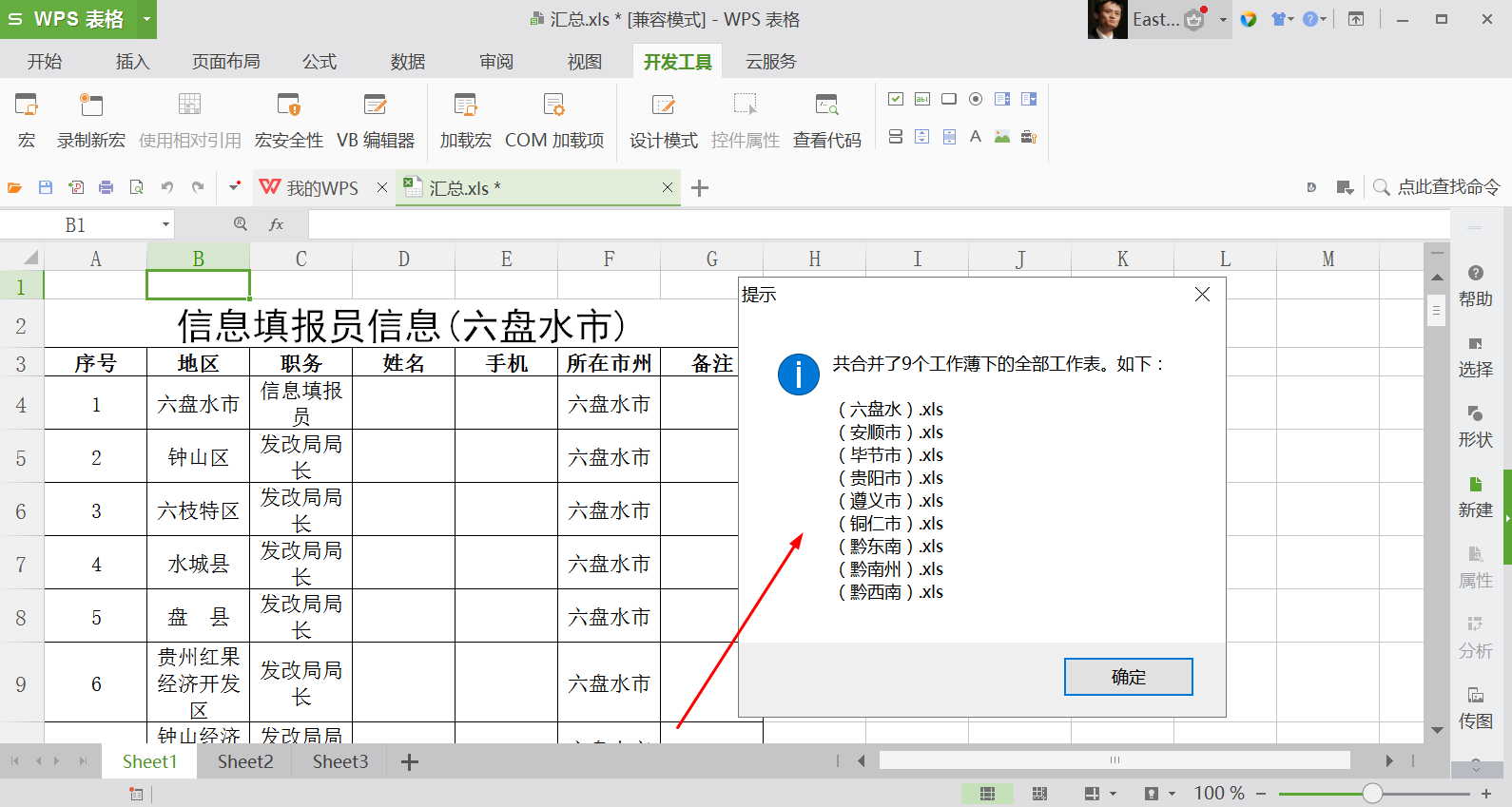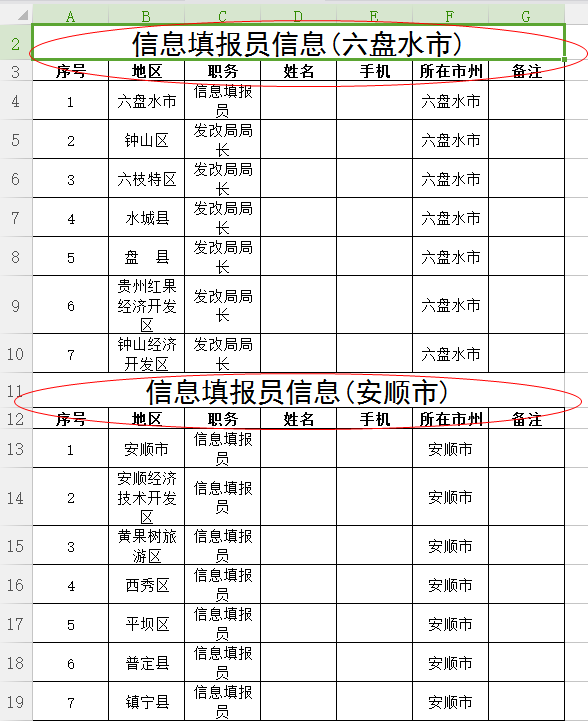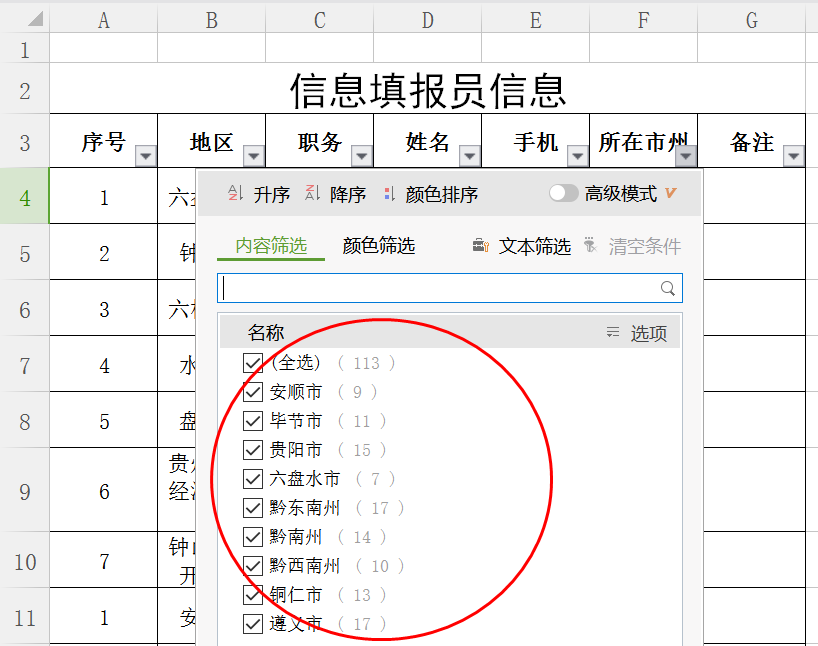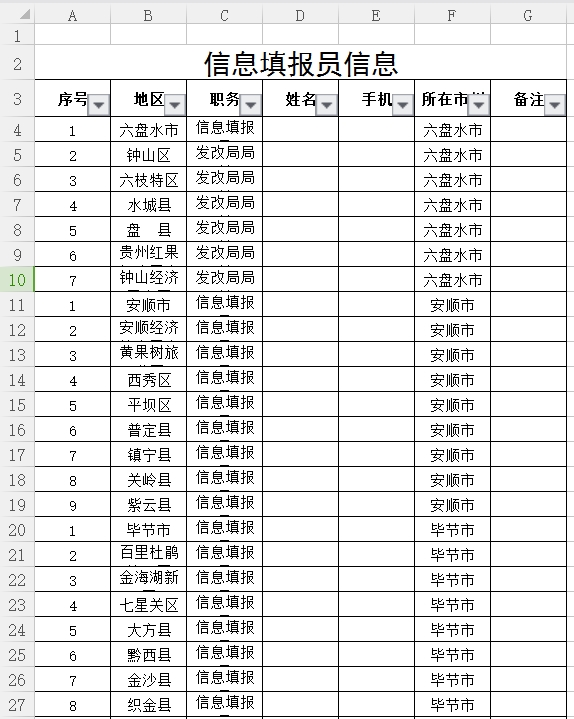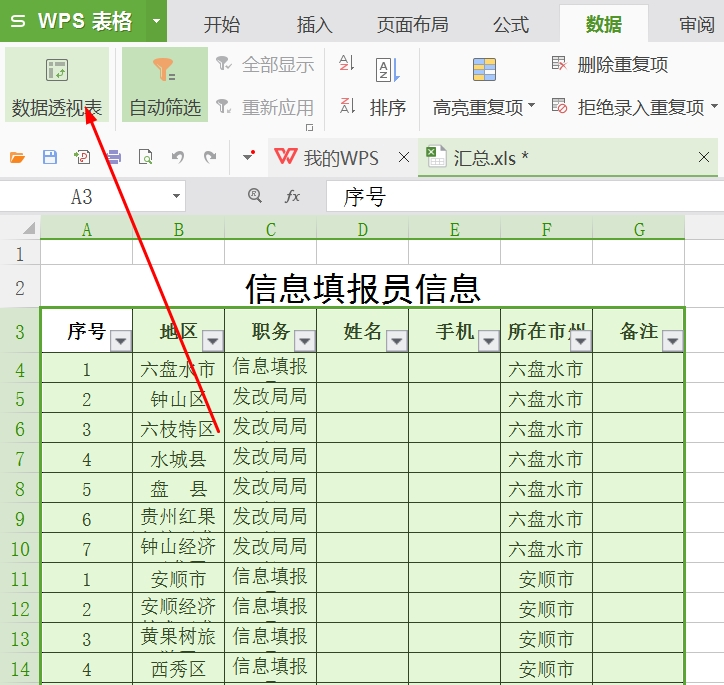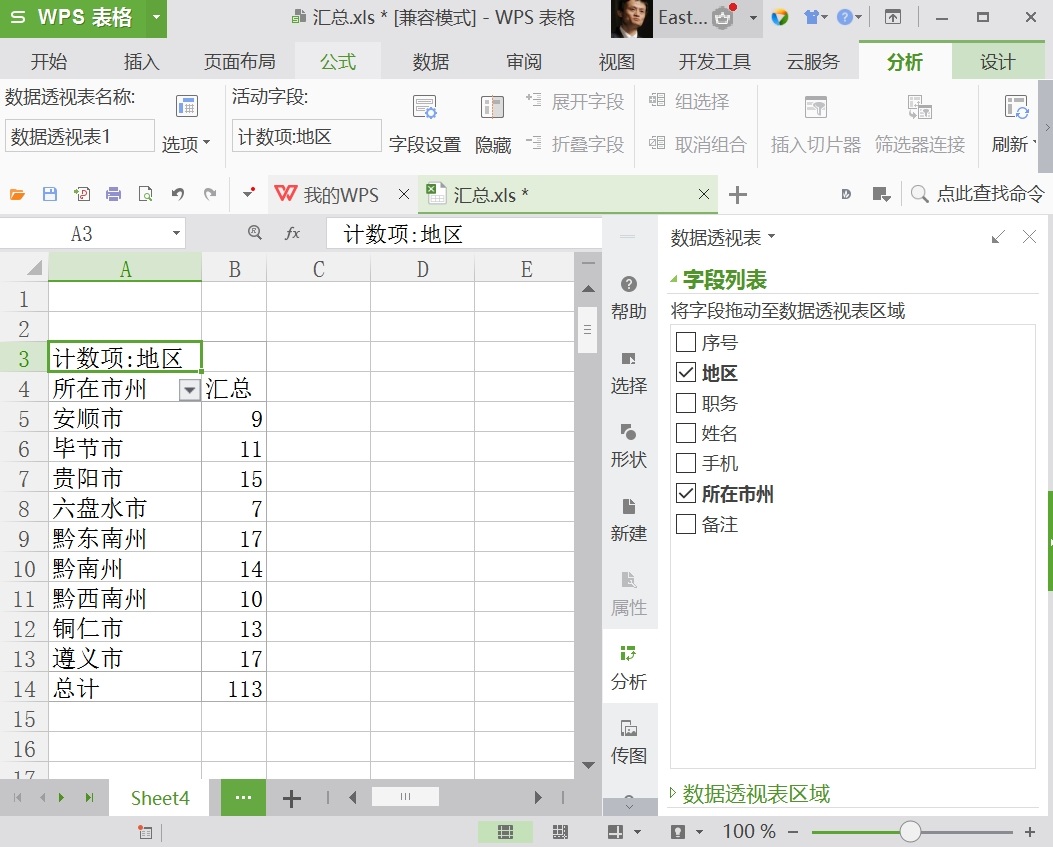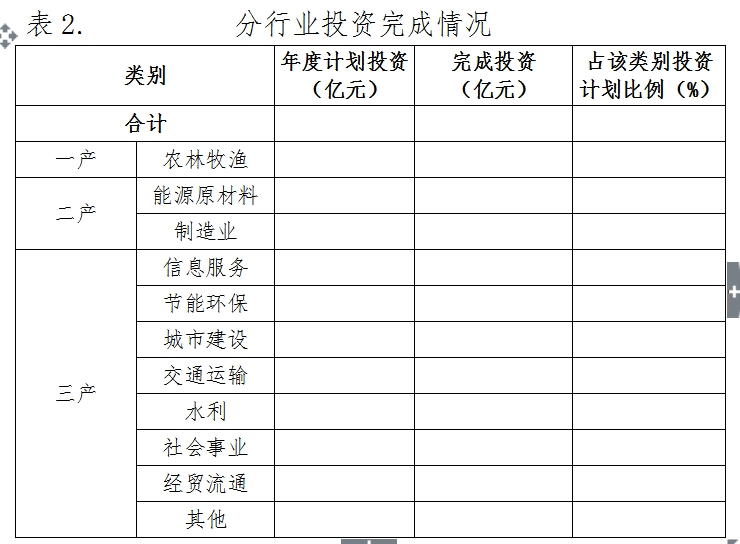二、合并当前文件各个子Sheet数据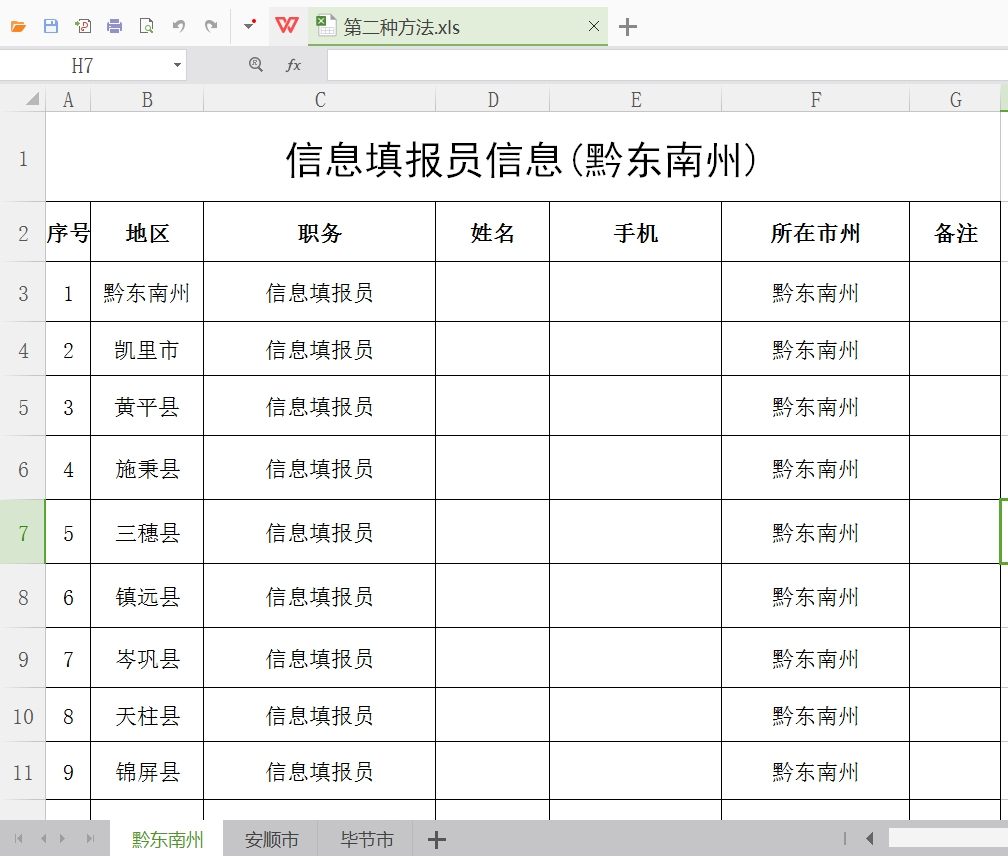Sub 合并当前工作簿下的所有市级分表工作表()
Application.ScreenUpdating = False
For j = 4 To Sheets.Count
If Sheets(j).Name <> ActiveSheet.Name
Then
X = Range("A65536").End(xlUp).Row + 1
Sheets(j).UsedRange.Copy Cells(X, 1)
End If
Next
Range("B1").Select
Application.ScreenUpdating = True
MsgBox "当前工作簿下的全部市级分表工作表已经合并完毕！", vbInformation, "提示"
End Sub


(By:Eastmount 2017-03-06 下午3点  http://blog.csdn.net/eastmount/ )